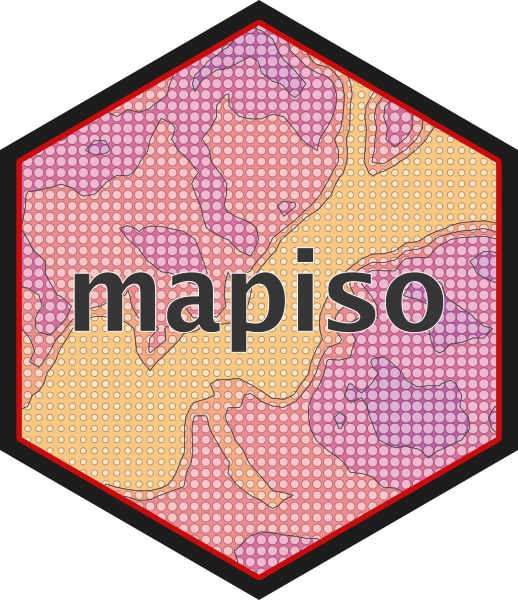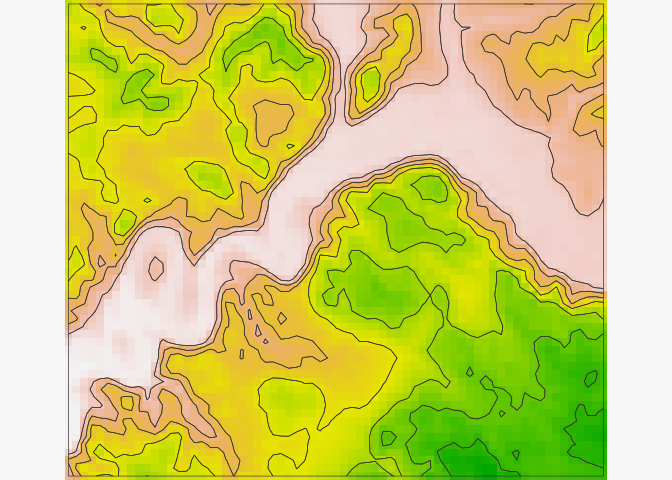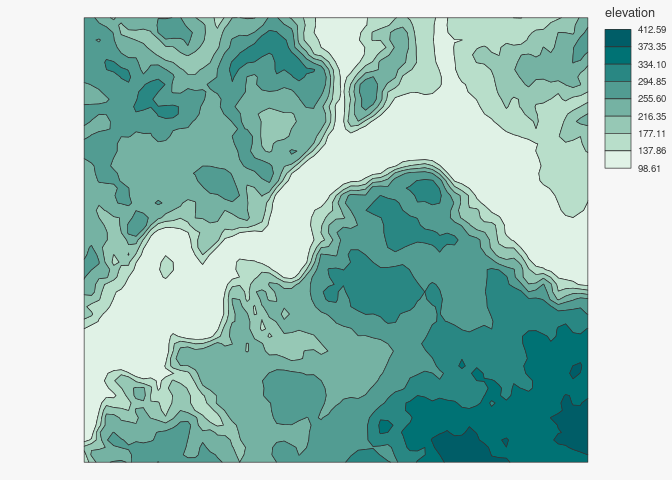# mapisoThe goal of `mapiso` is to ease the transformation of regularly spaced grids containing continuous data into contour polygons. These grids can be defined by data.frames (x, y, value), `sf` objects or SpatRasters from `terra`.
`mapsio` is a wrapper around `isoband`.

## Installation

You can install the released version of `mapiso` from CRAN with:

``install.packages("mapiso")``

Alternatively, you can install the development version of `mapiso` from GitHub with:

``remotes::install_github("riatelab/mapiso")``

## Usage

### Raster

``````library(mapiso)
library(terra)
#> terra 1.7.29
library(mapsf)
r <- rast(system.file("tif/elevation.tif", package = "mapiso"))
isor <- mapiso(x = r)
mf_theme(mar = c(0, 0, 0, 0))
mf_raster(r)
mf_map(isor, col = NA, add = TRUE)``````### sf regular grid

``````library(mapiso)
library(sf)
#> Linking to GEOS 3.9.0, GDAL 3.2.2, PROJ 7.2.1; sf_use_s2() is TRUE
library(mapsf)
# gridded data
s <- st_read(system.file("gpkg/elevation.gpkg", package = "mapiso"),
layer = "elevation", quiet = TRUE)
m <- st_read(system.file("gpkg/elevation.gpkg", package = "mapiso"),
layer = "com", quiet = TRUE)
# custom breaks
bks <-c(98,100, 150, 200, 250, 300, 350, 400, 412.6)
isos <- mapiso(x = s, var = "elevation", breaks = bks, mask = m)
mf_map(isos, "isomin", "choro",
breaks = bks, border = NA,
leg_title = "elevation")
mf_map(m, col = NA, add = TRUE)
mf_map(s, cex = 1, pch = ".", col = "grey20", add = TRUE)``````### data.frame

``````library(mapiso)
library(mapsf)
d <- read.csv(system.file("csv/elevation.csv", package = "mapiso"))
#>          x       y elevation
#> 1 586231.3 6431677  282.0299
#> 2 586481.3 6431677  263.7194
#> 3 586731.3 6431677  244.9597
#> 4 586981.3 6431677  222.3232
#> 5 587231.3 6431677  211.1522
#> 6 587481.3 6431677  232.1604
isod <- mapiso(x = d, var = 'elevation', coords = c('x', 'y'), crs = 'epsg:2154')
bks <- unique(c(isod\$isomin, isod\$isomax))
mf_map(isod, "isomin", "choro", breaks = bks, leg_title = "elevation")``````## Community Guidelines

One can contribute to the package through pull requests and report issues or ask questions here.
This project uses conventional commits and semantic versioning.